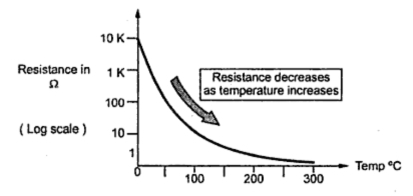### Conductivity Modulation

It is seen that the conductivity of semiconductors is directly proportional to the concentration of charge carriers. It can be increased by increasing n or p.
Note : The conductivity modulation is the controlled change in the conductivity of a semiconductors, in response to an external signal.
The increase in the charge carrier concentration can be achieved by many ways. The two important methods of increasing carrier concentration is semiconductors are,
1. By changing the temperature.
2. By illuminating the semiconductor with the focus of light.
By both the methods, new electron-hole pairs are generated and hence the conductivity can be controlled.
The method of changing the temperature is popularly used in thermistors while method of illumination is commonly used in photoconductors. Let us study these applications in detail.
1.1 Thermistos
The intrinsic concentration of an intrinsic semiconductor is given by,
And the conductivity of pure semiconductor is given by,
σi = nin + μp) q
It can be observed that as temperature increases, the intrinsic concentration increases and hence conductivity increases.
Note : The conductivity of Ge increases by about 6 % while that of Si by 8 % per degree increase in the temperature.
Due to such a large change in the conductivity with temperature, there is a limitation on the use of such semiconductor devices in some circuits.
But in some devices, such a property is advantageous. A devices which is based on this property is called thermistor.
Note : Thermistor is a device whose resistance depends on the temperature.
The silicon and germanium are very sensitive to the temperature and impurities and hence not directly used in thermistors. The practical thermistors use sintered mixtures of metalic oxides such as Nickel (NiO), Manganese (Mn2 O3), cabalt (Co2 O3), iron and uranium.
Thermistor is a contraction of  aterm 'thermal-resistors'. Thermistors is semiconductor device which behave as thermal resistors having negative temperature coefficient (NTC); i.e. their resistance decreases as temperature increases. The Fig. 1 shows this characteristic.Fig. 1 Resistance versus temperature characteristics of thermistor
The resistance of thermistor can be expressed as,
Where  RT : Resistance at T oK
R1  : Resistance at known temperature T1 oK
and       β = Characteristics temperature.
The thermistors are very sensitive and provide large change in resistance for small change in temperature. Typically it can provide a change of 80 ohms per degree celcius change in temperature.
In metals, as temperature increases, thermal vibrations of the ion increase. This disturbs the mean free path of the free electrons. This decreases their mobility and hence decreases the conductivity. Thus for the metals, the resistance increases by approximately 0.4 % / oC increases in temperature. Thus the metals have positive temperature coefficient. Hence by including a thermistor in a circuit, it is possible to compensate the effects of increases in temperature on the metals, over a range as wide as 100 oC.
Thermistors are available in a wide variety of shapes and sizes as shown in the Fig. 2.Fig. 1 Different forms of construction of thermistors

Smallest in size are the beads with a diameter of 0.15 mm to 1.25 mm. Beads may be sealed in the tips of solid glass rods to form probes. Disks and washes are made by pressing thermistor material under high pressure into flat cylindrical shapes. Washers can be placed in series or in parallel to increase power dissipation rating.
1. Small size and low cost.
2. Comparatively large change in resistance for a given change in temperature.
3. Fast response over a narrow temperature range.
4. Very much suitable for very precise temperature measurements and temperature compensations due to their high resolution.
1.1.2 Limitation of Thermistor
1. The resistance versus temperature characteristic is highly nonlinear.
2. Not suitable over a wide temperature range.
3. Because of high resistance of thermistor, shielded cables have to be used to minimize interference.
4. Requires Wheatstone bridge circuit and external power source for measurement.
1.1.3 Application of Thermistor
1. The thermistor's relatively large resistance change per degree change in temperature {known as sensitivity}makes it useful as temperature transducer.
2. The high sensitivity, together with the relatively high thermistor resistance that may be selected ( e.g. 100 kΩ), makes the thermistor ideal for remote measurement or control. Thermistor control systems are inherently sensitive, stable, and fast acting, and they require relatively simple circuitry.
3. Because thermistors have a negative temperature coefficient of resistance, thermistors are widely used to compensate for the effects of temperature on circuits performance.
4. Measurement of conductivity.
5. It is also used in thermometry, in the measurement of microwave- frequency power and as a thermal relay.
1.1.4 Sensistors
If a semiconductor is heavily doped, then it consists of large number of charge carriers. So it starts showing the properties of metal. Hence if temperature increases, such a heavily doped semiconductor shows increase in resistance due to decrease in the mobility of the change carriers. Such a device is called sensistor. Practically, the temperature coefficient of resistance is about + 0.7% /oC change in temperature, over the range from -60 oC to + 150 oC. The sensistors have positive temperature coefficient.